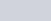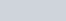The sum of digits of a two digit number is 13.
Question:

The sum of digits of a two digit number is 13. If the number is subtracted from the one obtained by interchanging the digits, the result is 45. What is the number?

Solution:

Let the digits at units and tens place of the given number be x and y respectively. Thus, the number is.

The sum of the digits of the number is 13. Thus, we haveAfter interchanging the digits, the number becomes.

The difference between the number obtained by interchanging the digits and the original number is 45. Thus, we have

$(10 x+y)-(10 y+x)=45$

$\Rightarrow 10 x+y-10 y-x=45$

$\Rightarrow 9 x-9 y=45$

$\Rightarrow 9(x-y)=45$

$\Rightarrow x-y=\frac{45}{9}$

$\Rightarrow x-y=5$

So, we have two equations

$x+y=13$

$x-y=5$

Here x and y are unknowns. We have to solve the above equations for x and y.

Adding the two equations, we have

$(x+y)+(x-y)=13+5$

$\Rightarrow x+y+x-y=18$

$\Rightarrow 2 x=18$

$\Rightarrow x=\frac{18}{2}$

$\Rightarrow x=9$

Substituting the value of in the first equation, we have

$9+y=13$

$\Rightarrow y=13-9$

$\Rightarrow y=4$

Hence, the number is $10 \times 4+9=49$.